# Simple Math

I ran across a puzzling math problem today. Subtracting a whole number from a fractional number does not give the correct answer. 10.4 - 10 = 0.4 BUT in SNAP! the answer is 0.4lotsofzeros36.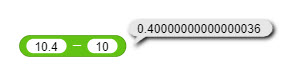I tried subtracting 10.0 instead, but get the same value.

I use Chrome on a PC.

Computers do not hold decimal numbers totally accurately so when you do this sort of maths - the inac curacies become apparent.

A solution I thought of was to multiply both operands by the same power of 10 to make the integers, doing the addition or subtraction, then dividing the result by the same power of 10. I haven't tested it yet, but I think it will work because I haven't seen the problem with addition and subtraction of integers. Now I have: https://snap.berkeley.edu/snap/snap.html#present:Username=snapenilk&ProjectName=alt%20add%20and%20sub

Oh, I'm happy -- I get to teach someone something!

You'll see the same problem when you do the division. The problem is that computers have only a finite memory. In the case of integers, that finiteness manifests itself in a limit to the size of integers you can represent. The arithmetic built into computer hardware has a relatively small limit, less than 100 factorial, but even with software arithmetic (as in our bignum library), there are only a finite number of atoms in the universe.

But with real numbers, or even just rational numbers, the problem is much worse, because those numbers are dense: there are infinitely many of them between any two of them. So, for example, there are infinitely many rational numbers between 0 and 1. There are infinitely many between 0.000000001 and 0.000000002. And so on. But there are only finitely many representations available in a computer. So, no matter what, almost all rational numbers can't be represented exactly in a computer. If you use the computer's hardware representation for real numbers, called floating point, then the only rational numbers that can be represented exactly are the ones whose denominator is a power of two.

For real numbers in general, that's the end of the story. But for rational numbers there's a better representation available: You represent the number as two integers, the numerator and the denominator. (If you want a unique representation, you require that the fraction be in lowest terms, i.e., that the numerator and denominator have no common factor larger than 1. And that the denominator be positive.) Then every rational number can be represented exactly, up to the limit on representability of integers, namely, the numerator and denominator can't be too big. The same library that gives us extra-large integers (the feature is called "infinite precision" but of course it's not really infinite) also gives us exact rationals. So the problem the OP posed isWhat's more, if you use exact rationals inside the computer, you can give an exact decimal representation (0.4) externally if you generate it as a character string, not as a number. (Of course if the fraction is 1/3 then you can't generate an exact decimal representation no matter how you go about it.)

So, since there are only finitely many floating point representations, each of them actually represents a range of numbers. The floating point representation that is displayed as 0.40000000000000036 represents a range of values that includes 4/10. But there's only one number that it represents exactly, and that's 0.40000000000000036.

We could look at the decimal representation of a value, treat it as a character string, and look for long substrings of zeros (or nines, if the value exactly represented in floating point is a little less than the value we want), and manipulate the digits as text to get a rounded version. (That's sort of what variable watchers do, since if you put 0.40000000000000036 in a variable, its watcher shows 0.4. But I think it's just rounding to the nearest some-small-number-of digits, rather than checking the characters in the string.)

So far we are only talking about ugly representations seen by users, but not actual program bugs. Those come in when you do equality comparisons: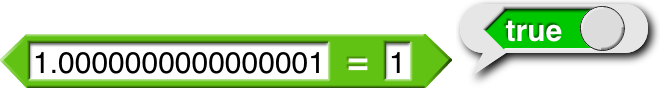Depending on what your program is going to do based on the result of the comparison, this could result in horrible misbehavior.

They make you learn all this stuff when you major in computer science, but honestly, floating point computation is one of the two things (the other is security) that I think you should never try to program yourself, but instead hire a specialist. In cases where your program really matters, I mean, of course; knock yourself out experimenting with these ideas.

Good Golly, Brian, you do go in for completeness!Much of this can be kind of ignored by truncation (specification of number of digits to maintain; not the same as rounding) but I did not see a trunc function. Is there something in Snap! that can be set to specify precision? I did not recognize this is Settings, and I haven't found a function/operation that purports to do this.

I went down this rabbit hole when I was trying to separate a value into an integer and it's decimal part. I don't have a project, but was just doodling around.

I just tested my solution and it works.

Huh. Right you are. I don't understand why, though... I'm going to have to think harder about this.

In the pulldown menu of the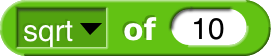block, you'll find floor and ceiling.

We don't have a precision setting. I was taught that that's a wrong approach, that every so often you'll get really strange results if you just truncate. Although I suppose we could keep full precision internally and just truncate for display purposes, as the variable watchers do.

But why don't you want round? If the value you have is 3.99999999999997, taking the floor is the wrong thing.

I think the really right thing is to examine the digits of the numeral and recognize the case of a bunch of consecutive zeros or nines, and only then round. To take a somewhat artificial example,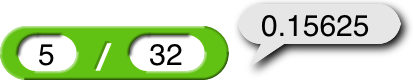should really show all five significant digits. And even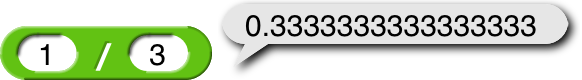benefits pedagogically from showing a lot of digits.

But why does @snapenilk's algorithm work? What that tells me is that 0.40000000000000036 isn't the closest representable value to 0.4. So why doesn't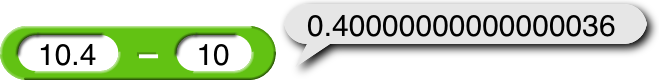give 0.4?

Oh, wait, when you subtract 10 from 10.4, the normalization process left-shifts the answer so that the most significant bit is 1. Whereas @snapenilk's version doesn't result in a denormalized intermediate form. So I guess I have to look at the internal floating point actual bits to understand what it's doing... Ugh...

@bh do you know the more common name for one trio-trigintillion?
[poll type=regular results=on_vote public=true chartType=bar]

• nothing
[/poll]

Nope, I've never heard of a trigintillion so have no hope of working it out.

it's about 30-illion if a million was 1-illion and a billion was 2-illion

First of all, Google is a search engine, not a number.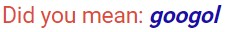Secondly, googol has

one hundred zeros (one hundred one decimal places), which is called:
Ten duotrigintillion.
https://keelyhill.github.io/tuppers-formula/

you are correct!

for snapenilk only

haha! snapenilk has already seen this

Now that I saw it, you can delete it so that nobody else can see it.

now look

The circle is complete now! What started me down this hole was playing with the floor and ceiling functions.

I've implemented my own training-wheels version of floating point in an old project when it mattered, but the REAL take-away here is to beware of using equality in tests. GT or LT might be better.